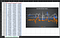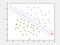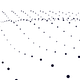# A Simple Guide for Sign Language Classification Using Support Vector Machines

With the rapid growth in the Machine Learning(ML) field in the recent past, many powerful algorithms have emerged to solve various classification/regression problems and to mimic the human brain activity. In this article we will be looking at data preprocessing, feature selection and classification of some gestures in the American Sign Language(ASL) using Support Vector Machines(SVMs). I will be using Python as the programming language for this process. In addition to the code let’s try to understand the procedure so that it’ll help you to implement it in any other programming language you prefer.

We will be using data from the Myo armband dataset for some American Sign Language Signs which consist of Electromyography(EMG) signals from 8 EMG sensors and signals from an Inertial Measurement Unit(IMU) placed in the MYO Armband. This dataset consist of 20 classes and data taken from 9 users. A single .csv file represents a single class and the content in a single file is explained here. Since these files consist of time-series data points, using these data as it is will not get us anywhere in the classification process. The two images below visualizes the signal data taken from one MYO Armband.Left: A Plot of EMG signals , Right: A plot of Accelerometer signals

From the above images it is clear that the recorded data will not make sense to classify any gesture. That’s why converting data to represent a class in a more sensible manner is vital in any machine learning problem. That’s where data preprocessing comes into play. The features you compute out of the data should be a fair representation of the original data that belongs to a class. Let us now dive into the data preprocessing stage.

To represent the characteristics of the original signal it is important to select a balanced set of features that will represent both the energy and frequency aspects of the signal. The selected features are:

• Mean Absolute Value (MAV)- This gives information on muscle contraction levels and also it provides robustness to noise.
• Root Mean Square (RMS)- It reflects the mean power of the signal and is related to the constant force and non-fatiguing contractions.
• Variance (VAR)- A measure of the power density of a signal (x bar denotes the mean of the samples in a column)
• Simple Square Integral (SSI)- It gives a measure of the energy of the EMG signal.
• Maximum value (MAX) and Minimum value (MIN)- These two features are mainly used to represent the characteristics of the three axes of the accelerometer and gyroscope readings
• Zero Crossing (ZC)- It is the number of times the waveform crosses zero. This feature provides an approximate estimation of frequency domain properties.

x: a sample value, N = 50 for each case above.

All the above features should be calculated for each sensor reading available in the data set. There are 8 EMG sensor readings, 3 accelerometer readings, 3 gyroscope readings and 3 roll, pitch and yaw readings per arm. Since this data set utilizes both arms there will be (2 x (17)) 34 sensor readings per .csv file. Since the roll, pitch and yaw readings are always positive readings we will not calculate the ZC rate for those readings. Therefore, the total number of calculated features will be 232 features. Below are some code snippets to calculate each feature.

A sample of the processed data is shown in the figure below:

What we can observe is that, the features of a single example are of different scales. Therefore, we cannot use these examples as it is for an SVM model since the features which are within a larger scale will tend to dominate other features and it may slow the training process. This is why feature scaling is considered to be important during data preprocessing. Fortunately the preprocessing library of scikit-learn facilitates feature scaling. We will be using the MinMaxScaler since our data set is not following a gaussian distribution and by default it will scale the features within the range [0,1]. Let’s take a look at the code below for a better understanding as to how this should be done.

Due to the limited number of examples (only 550 examples in total) in the dataset, this large feature set is more prone to overfit any model we might plan to train. So how can we reduce the number of features without affecting the characteristics of the original signal? That’s where feature selection comes in to play. Manually selecting the most important features out of 232 features is going to take a long time. That’s when algorithms such as Random Forest comes to the rescue and python’s scikit-learn conveniently offers a `RandomForestClassifier` . Random Forest is an elegant algorithm that can be used in classification problems and for feature selection. It will classify its examples using the features with the highest importance towards grouping the examples into classes . This means that it will not make use of all the features for classification. Therefore, we can use this property of Random Forest to extract features of the highest importance for training purposes.

For more in-depth explanation on Random Forest you can refer this article:

First we will have to split our dataset into train and test sets. The splitting of data should be done in a random manner. Therefore, we will be using train_test_split from the model_selection library in scikit-learn:

Once that’s done we will be able to perform feature selection: (for more information on the classifier used in python visit here)

The get_support() method returns an array of boolean values, where the TRUE values are features whose importance is greater than the mean importance. Now you are ready to train an SVM model.

SVM is a powerful model used in the analysis of data for classification problems. It will construct a decision boundary/boundaries (also known as hyperplanes) in 2D or high dimensional space which will separate the training data into separate classes such that the distance to the nearest data point of a class is maximized. The SVM model tries to give the best decision boundary out of the many possible decision boundaries by maintaining a large margin to the classes on either side of the boundary to reduce the generalization error. Due to their behavior they are also called large margin classifiers.Linear classification of data using a SVM model. The blue line represents the decision boundary

SVMs are capable of performing linear classifications as well as non-linear classifications using kernels. We will be using a gaussian kernel for this classification. The Support Vector Classifier (SVC) in the SVM library of scikit-learn will be used for our purpose. We will have to specify three parameters for this classifier. They are; the kernel type, C and gamma values. The ‘rbf’ or gaussian kernel will be used as mentioned before. C is a parameter in the error function, that should be defined to train the model. Here we use C = 10. Gamma is the kernel coefficient and we have equated it to 1. Now let’s see how the training will be done using python:

The optimum C and gamma parameters can be selected using `GridSearchCV` in the scikit-learn model_selection library. We will be passing a set of C and gamma parameter values as arguments to the support vector classifier and GridSearchCV will build a model for every combination of parameters and then evaluate each model, so that the best parameters will be retained. We can then extract the parameters as shown in the print() statement below:

With this you have completed training your model 😄 !

Finally we will be feeding our model unseen data, also known as test data, to see the performance, or in other words, to see how it would generalize to unseen data. The code snippet below will make predictions using the test data set:

`y_pred = svclassifier.predict(X_test)`

Once you’ve done that, how will you know the results? The scikit-learn package has a metrics library which can be used for our purpose. From that library we can obtain a confusion matrix and a classification report. A confusion matrix can be easily displayed using a heat map. We can use the famous data visualization library- seaborn and the matplotlib library in python to create a heat map. We can visualize the confusion matrix as follows:

Your confusion matrix will look similar to the following:

We can simply display the classification report as follows:

`print(classification_report(y_test,y_pred))  # y_test: the actual labels# y_pred: the predicted labels`

The confusion matrix and the classification report shows that our model is able to perform classification with a high accuracy(~93%). I hope these techniques I introduced gave you a better understanding on how to build a classification model using a limited amount of time series data.

Written by

Written by

#### A third year Electronic and Telecommunication Engineering undergraduate who loves music, reading and learning new concepts in machine learning and robotics